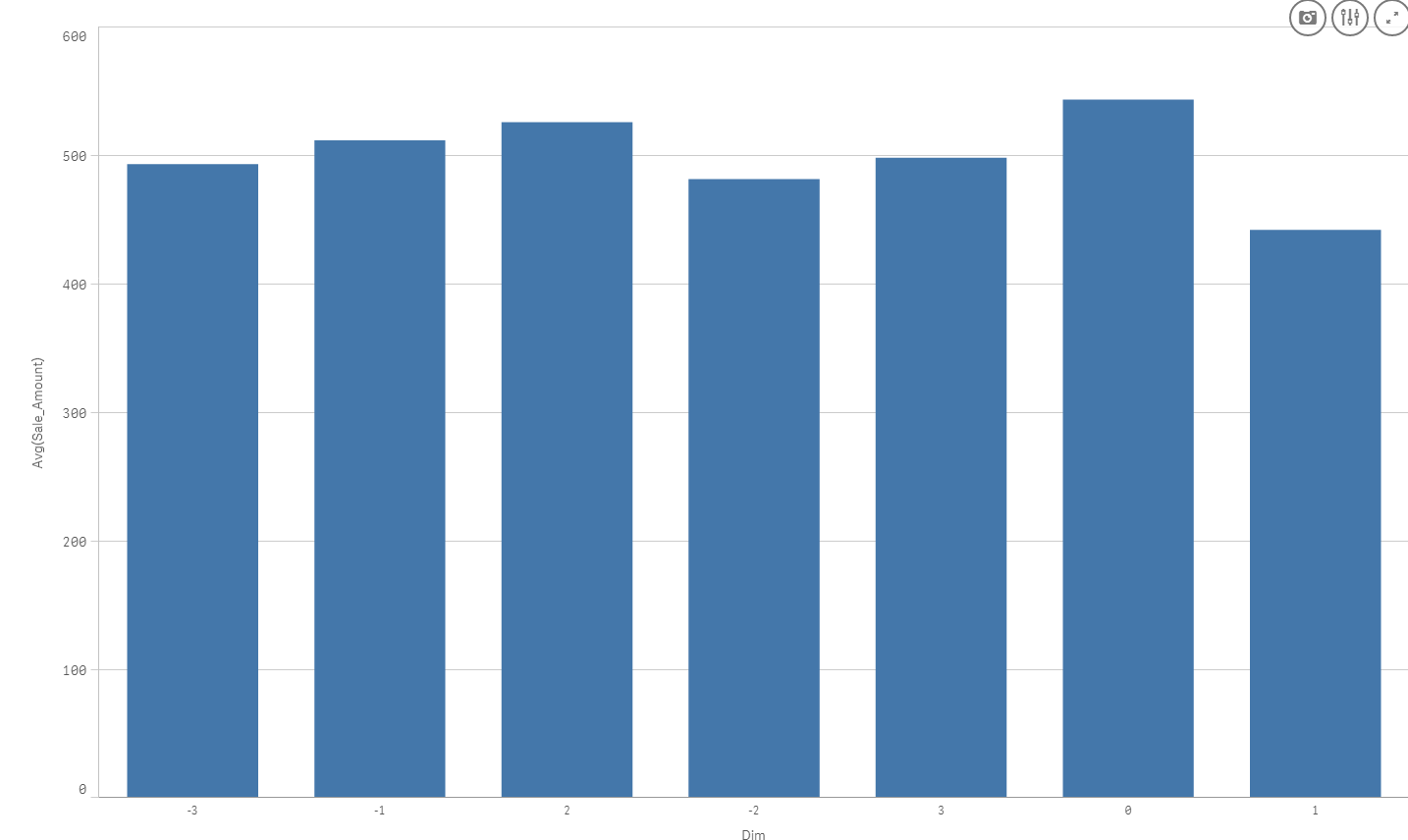Announcements
MAINTENANCE ALERT: Dec. 12th starting 9 AM CET. Community will be read-only. GET DETAILS
cancel
Showing results for
Did you mean:Contributor II

## Calculated Dimension

Can someone explain why a simple calculation does not work when calculating a dimension?

• if (max(BILL_DATE)>Today(),'T','F')

The expression is OK, although it creates a invalid Dimension.

Thank you,

1 Solution

Accepted SolutionsMVP

Try this:

Aggr(if (\$(vLastTransaction) < 8 ,3,

if (\$(vLastTransaction) >= 8 and \$(vLastTransaction) < 15 ,2,

if (\$(vLastTransaction) >= 15 and \$(vLastTransaction) < 22 ,1,

if (\$(vLastTransaction) >= 22 and \$(vLastTransaction) < 29 ,0,

if (\$(vLastTransaction) >= 29 and \$(vLastTransaction) < 60, -1,

if (\$(vLastTransaction) >= 60 and \$(vLastTransaction) < 90 ,-2,

if (\$(vLastTransaction) >= 90 ,-3,'N/A'))))))), Customer)5 RepliesMVP

If(Max(TOTAL BILL_DATE) > Today(), 'T', 'F')MVP

or you can do this:

Aggr(If(Max(BILL_DATE) > Today(), 'T', 'F'), BILL_DATE)Contributor II
Author

Thank you Sunny both options worked! Does this mean only aggregated type formulas work in the dimension?

I have a more complex example where I am generating a recency score by customer. The formula works below in a measure within a table by customer. Although when I try to use the recency score as a dimension it does not work.

See sample app attached.

 Variable # of Days since last transaction Max Date of Data Set minus Max Date per Customer vLastTransaction MAX(total{=0<=11"}, BILL_TYPE_DESC = {"Invoice"} >}BILL_DATE) - MAX({=0<=11"}, BILL_TYPE_DESC = {"Invoice"} >} BILL_DATE) Formula to assign Recency Score Formula Comment if [\$(vLastTransaction)] < 8 ,3, //Score 3 : if last transaction less than 1 week if [\$(vLastTransaction)] >= 8 and [\$(vLastTransaction)] < 15 ,2, //Score 2 : if last transaction within 2 weeks if [\$(vLastTransaction)] >= 15 and [\$(vLastTransaction)] < 22 ,1, //Score 1 : if last transaction within 3 weeks if [\$(vLastTransaction)] >= 22 and [\$(vLastTransaction)] < 29 ,0, //Score 0 : if last transaction within 4 weeks if [\$(vLastTransaction)] >= 29 and [\$(vLastTransaction)] < 60, -1, //Score -1 : if last transaction within 2 months if [\$(vLastTransaction)] >= 60 and [\$(vLastTransaction)] < 90 ,-2, //Score -2 : if last transaction within 3 months if [\$(vLastTransaction)] >= 90 ,-3,'N/A'))))))) //Score -3 : if last transaction greater 3 monthsMVP

Try this:

Aggr(if (\$(vLastTransaction) < 8 ,3,

if (\$(vLastTransaction) >= 8 and \$(vLastTransaction) < 15 ,2,

if (\$(vLastTransaction) >= 15 and \$(vLastTransaction) < 22 ,1,

if (\$(vLastTransaction) >= 22 and \$(vLastTransaction) < 29 ,0,

if (\$(vLastTransaction) >= 29 and \$(vLastTransaction) < 60, -1,

if (\$(vLastTransaction) >= 60 and \$(vLastTransaction) < 90 ,-2,

if (\$(vLastTransaction) >= 90 ,-3,'N/A'))))))), Customer)Contributor II
Author

THANK YOU!!!  That workedCommunity Browser